# How to Calculate and Solve for Bulk Density | Soil Mechanics and Foundation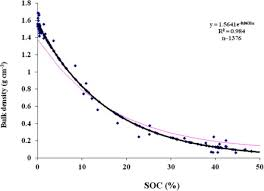The image above represents bulk density.

To compute for bulk density, two essential parameters are needed and these parameters are Mass of the soil (m) and Volume of the soil (V).

The formula for calculating bulk density:

sb = m / V

Where:

sb = Bulk Density
m = Mass of the Soil
V = Volume of the Soil

Let’s solve an example;
Find the bulk density when the mass of the soil is 24 and the volume of the soil is 6.

This implies that;

m = Mass of the Soil = 24
V = Volume of the Soil = 6

sb = m / V
sb = 24 / 6
sb = 4

Therefore, the bulk density is 4.

Calculating the Mass of the Soil when the Bulk Density and the Volume of the Soil is Given.

m = sb x V

Where;

m = Mass of the Soil
sb = Bulk Density
V = Volume of the Soil

Let’s solve an example;
Find the mass of the soil when the bulk density is 10 and the volume of the soil is 4.

This implies that;

sb = Bulk Density = 10
V = Volume of the Soil = 4

m = sb x V
m = 10 x 4
m = 40

Therefore, the mass of the soil  is 40.

Calculating the Volume of the Soil when the Bulk Density and the Mass of the Soil is Given.

V = m / sb

Where;

V = Volume of the Soil
sb = Bulk Density
m = Mass of the Soil

Let’s solve an example;
Find the volume of the soil when the bulk density is 15 and the mass of the soil is 45.

This implies that;

sb = Bulk Density = 15
m = Mass of the Soil = 45

V = m / sb
V = 45 / 15
V = 3

Therefore, the volume of the soil is 3.

Nickzom Calculator – The Calculator Encyclopedia is capable of calculating the bulk density.

To get the answer and workings of the bulk density using the Nickzom Calculator – The Calculator Encyclopedia. First, you need to obtain the app.

You can get this app via any of these means:

To get access to the professional version via web, you need to register and subscribe for NGN 2,000 per annum to have utter access to all functionalities.
You can also try the demo version via https://www.nickzom.org/calculator

Apple (Paid) – https://itunes.apple.com/us/app/nickzom-calculator/id1331162702?mt=8
Once, you have obtained the calculator encyclopedia app, proceed to the Calculator Map, then click on Agricultural under Engineering.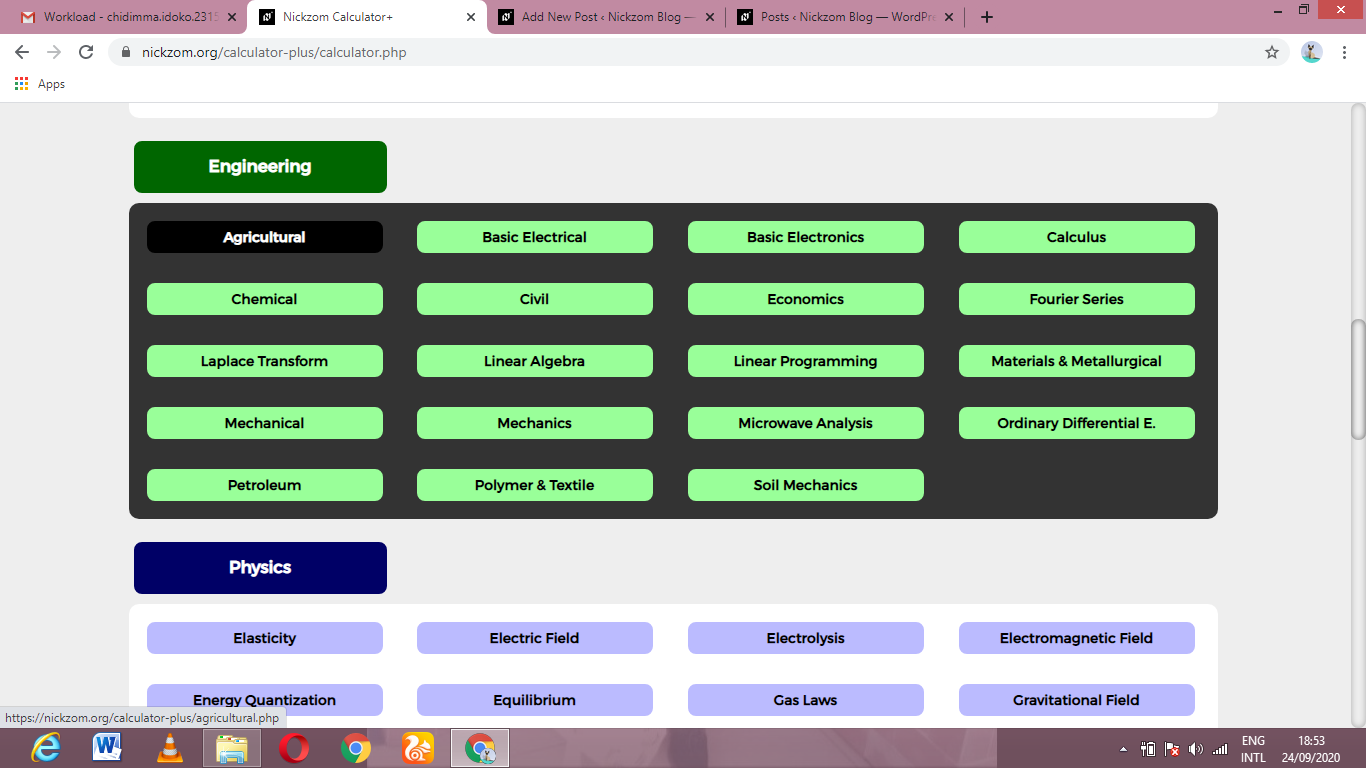Now, Click on Soil Mechanics and Foundation under Agricultural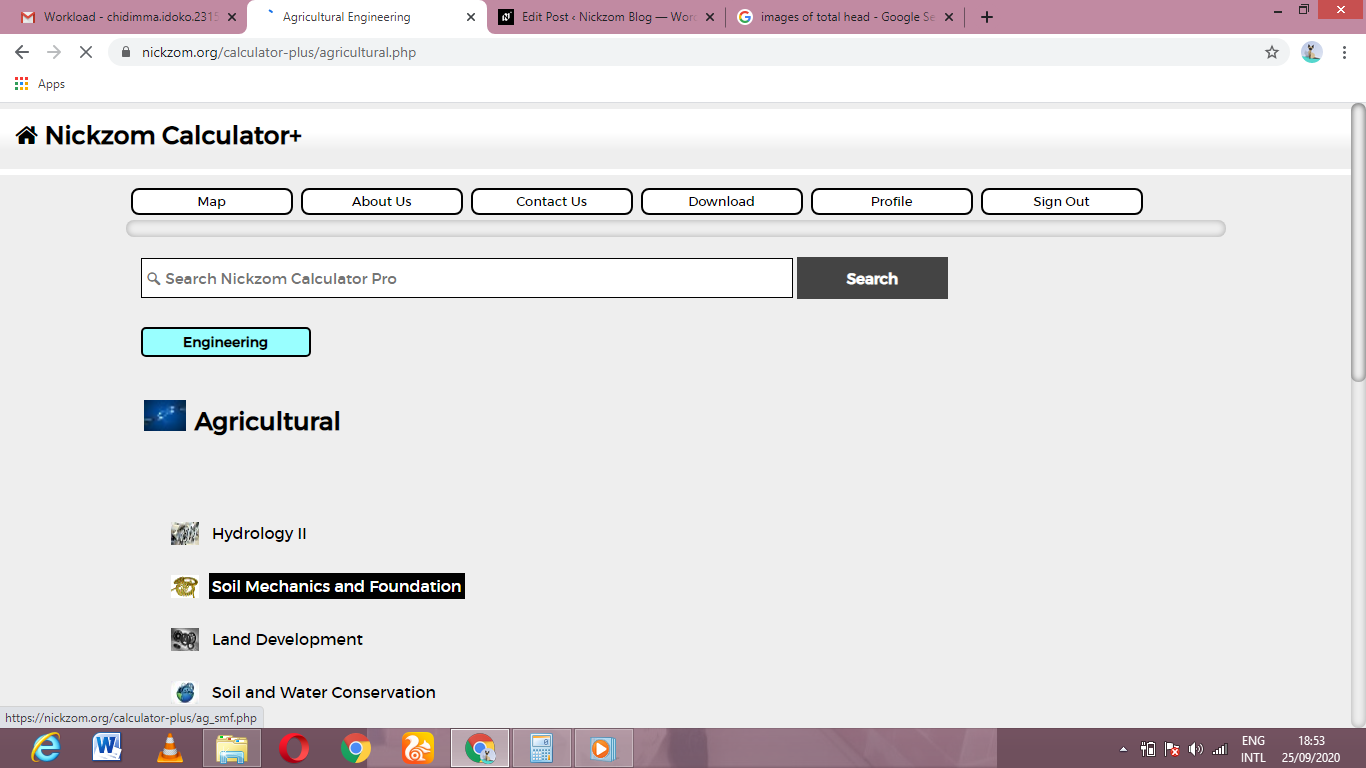Now, Click on Bulk Density under Soil Mechanics and Foundation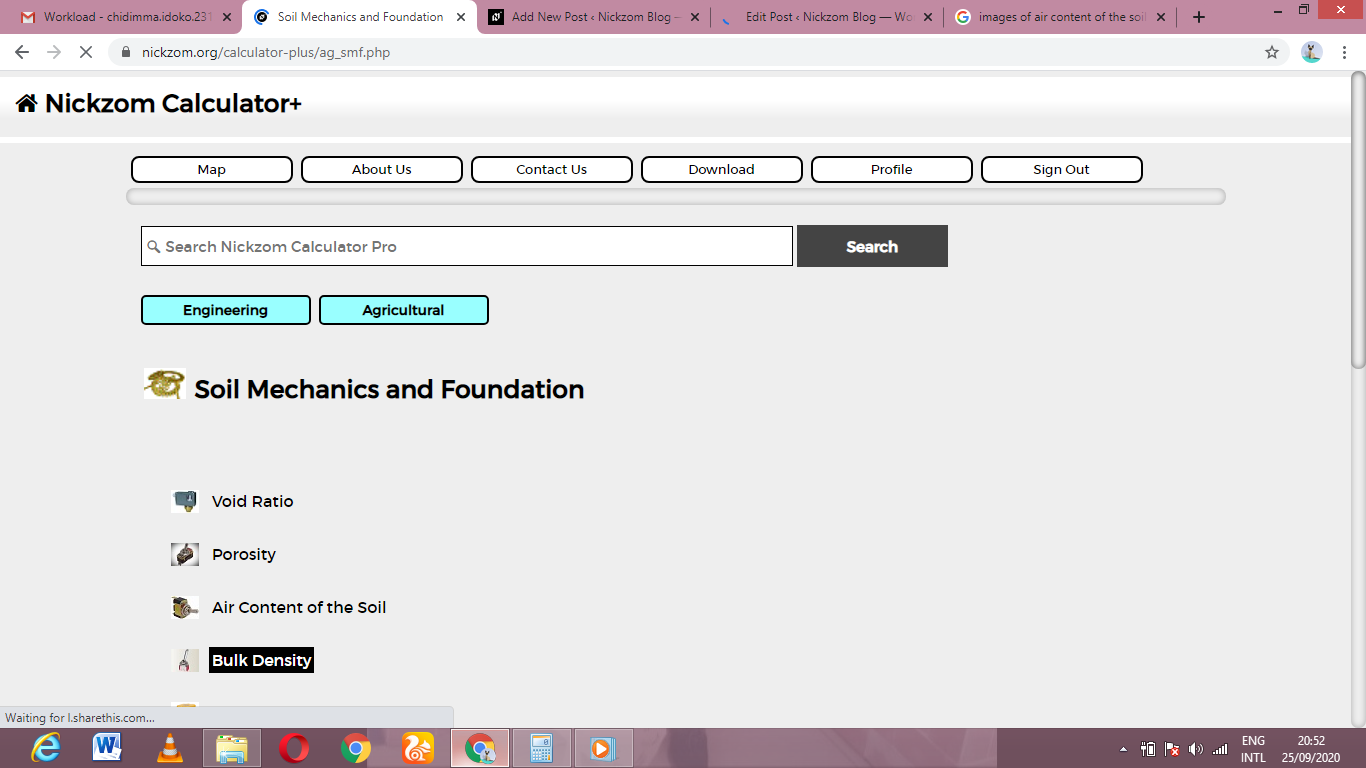The screenshot below displays the page or activity to enter your values, to get the answer for the bulk density according to the respective parameters which is the Mass of the soil (m) and Volume of the soil (V).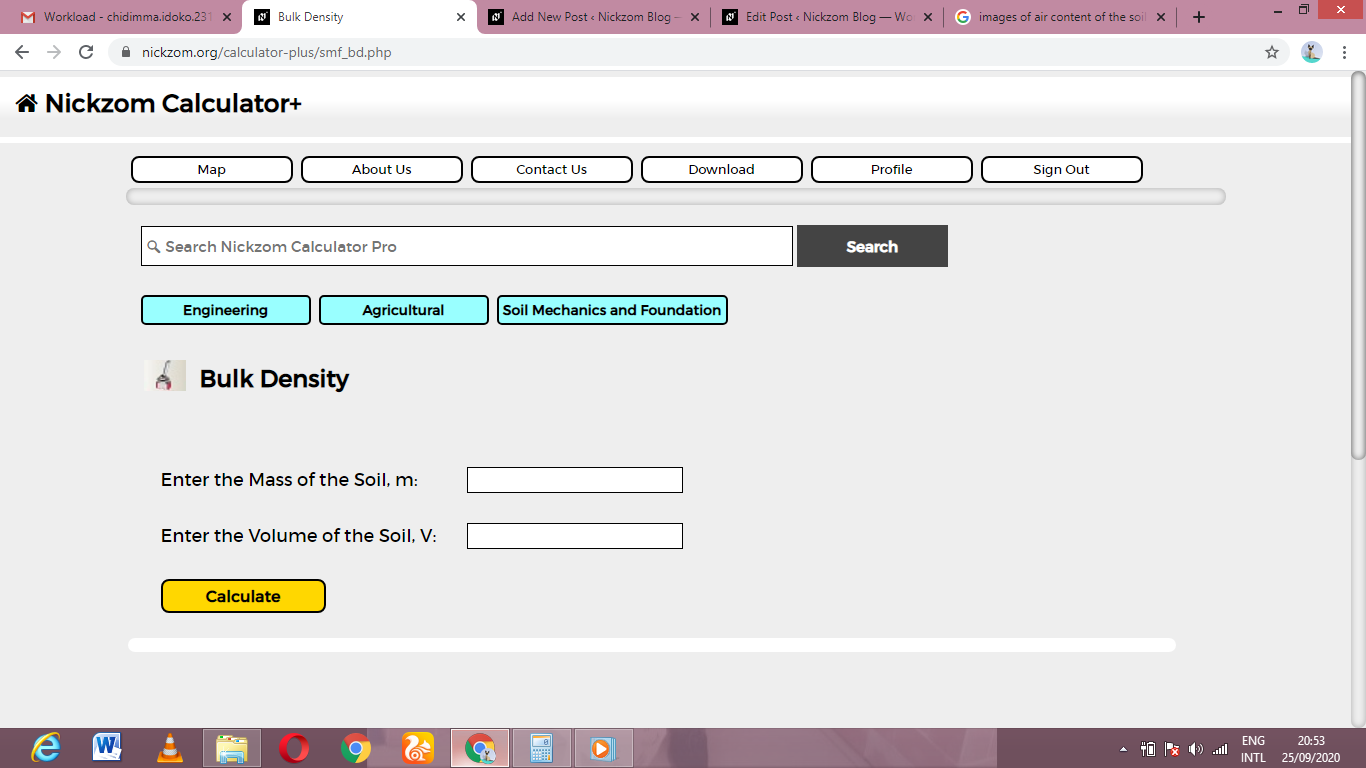Now, enter the values appropriately and accordingly for the parameters as required by the Mass of the soil (m) is 24 and Volume of the soil (V) is 6.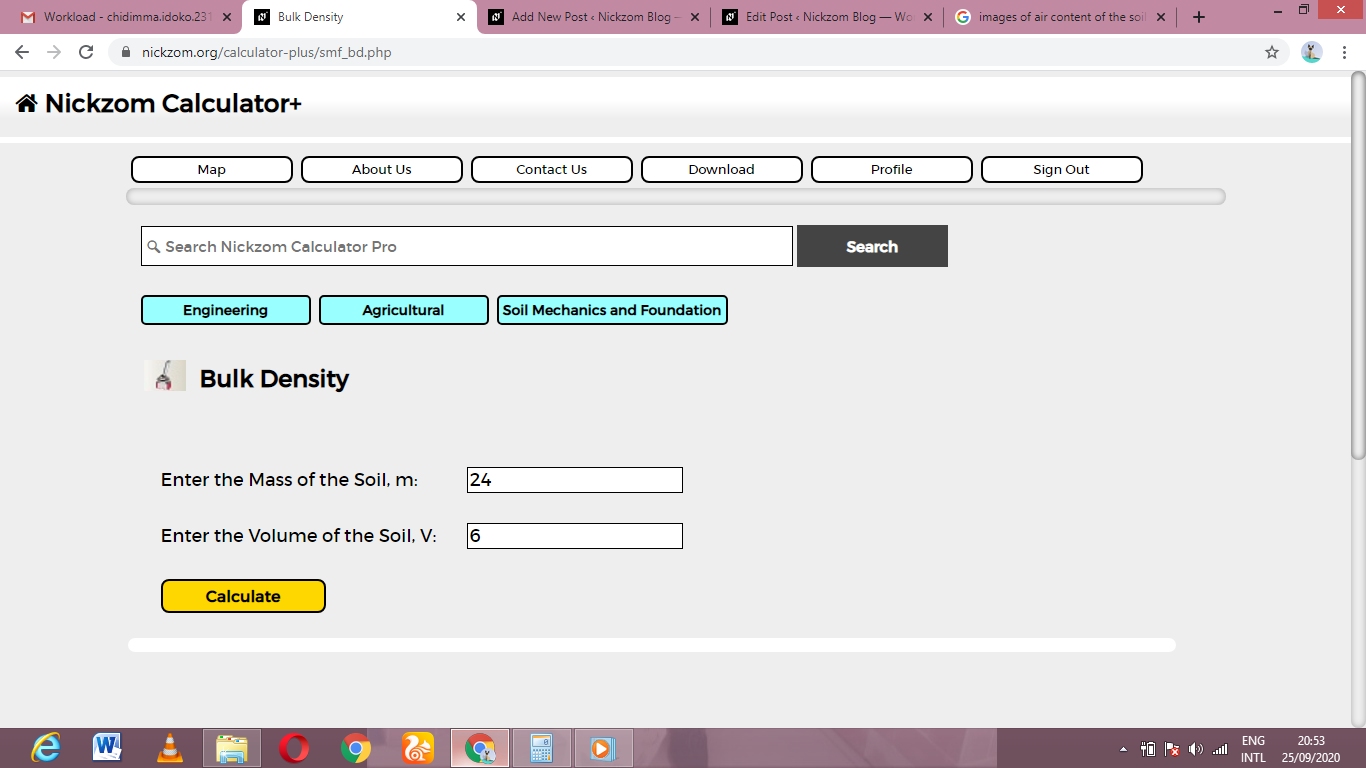Finally, Click on Calculate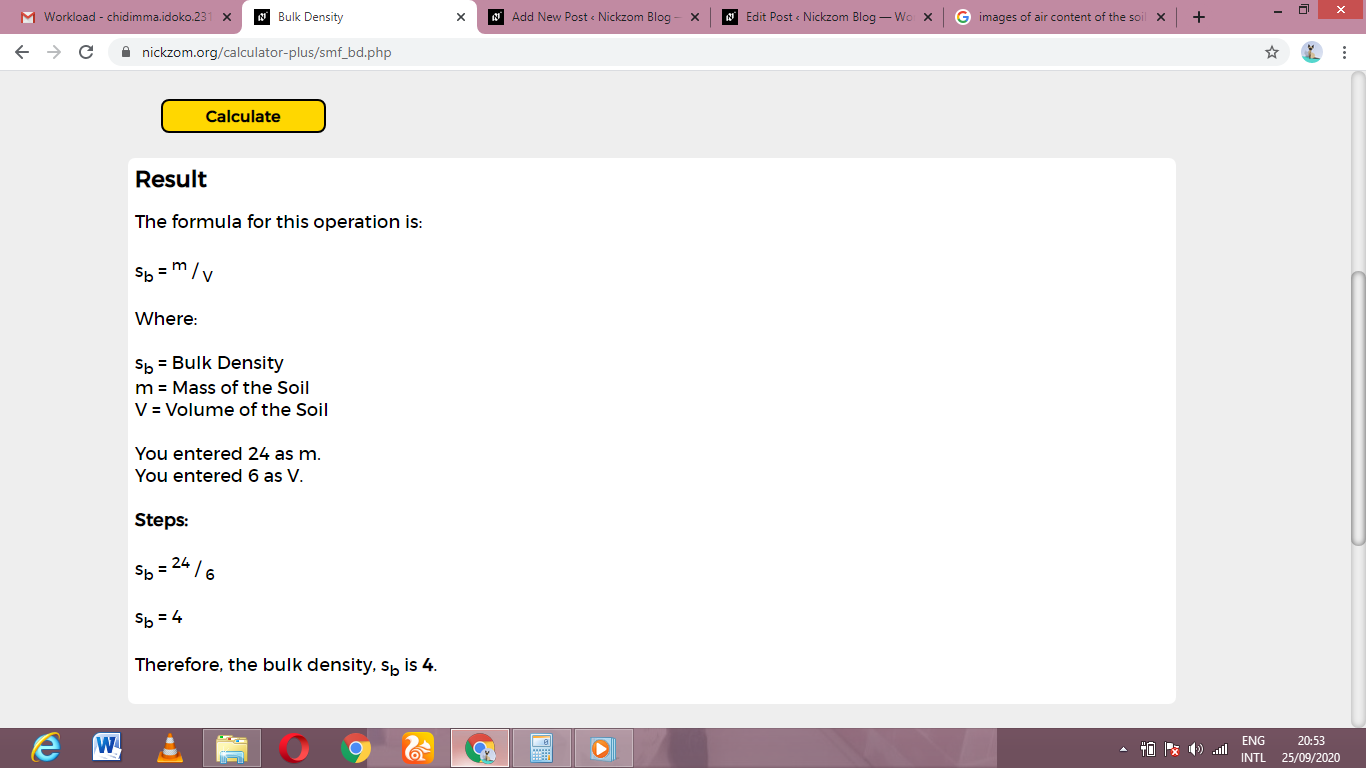As you can see from the screenshot above, Nickzom Calculator– The Calculator Encyclopedia solves for the bulk density and presents the formula, workings and steps too.